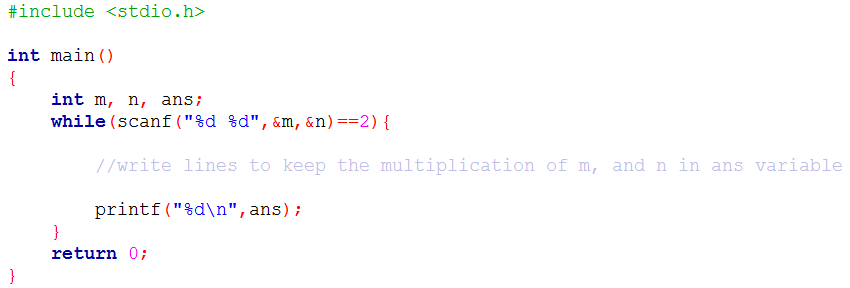Problems
10022 - Multiplication

Multiplication

Time Limit: 1 sec

The Problem

In this problem, you will be given 2 integer numbers m, n as input. Your job is to print the multiplication of m and n.

Your code might look like below:The Input

There are several lines of input in the input file. Each line contains two integer numbers m and n (absolute value of m, and n will be less than 100). Input is terminated by EOF.

The Output

For each line of input you have to print a line of output, this line contains an integer which is the multiplication value of m and n.

Sample Input

2 10

6 -3

Sample Output

20

-18

Online Mock Contest 2016

Problem Setter: Shahin ShamS